# Intermediate Maths Solutions for exercise 6(d) Trigonometric Ratios upto Transformations

Inter maths solutions for Trigonometric Ratios upto Transformations exercise 6(d) are given.

Thsese are very easy to understand. Please study the text book lession very well. Then observe the example problems and solutions.

Observe the given solutions and try them in your own method.

You can see the solutions for Intermediate first year maths text book.

You can see the text book solutions for

Functions

Exercise 1(a)

Exercise 1(b)

Exercise 1(c)

Mathematical Induction

Exercise 2(a)

Matrices

Exercise 3(a)

Exercise 3(b)

Exercise 3(c)

Exercise 3(d)

Exercise 3(e)

Exercise 3(f)

Exercise 3(g)

Exercise 3(h)

Exercise 3(i)

Exercise 4(a)

Exercise 4(b)

Product of Vectors

Exercise 5(a)

Exercise 5(b)

Exercise 5(c)

Trigonometric Ratios upto Transformations

Exercise 6(a)

Exercise 6((b)

Exercise 6(c)

Exercise 6(d)

Exercise 6(e)

Exercise 6(f)

Trigonometric Equations

Exercise 7(a)

Inverse Trigonometric functions

Exercise 8(a)

Hyperbolic Functions

Exercise 9(a)

Properties of Triangles

Exercise 10(a)

Exercise 10(b)

Inter Maths Trigonometry text book solutions

Maths 1b

Locus

Exercise 1(a)

Transformations of axes

Exercise 2(a)

Three Dimensional Coordinates

Exercise 5(a)

Exercise 5(b)

Direction Cosines and Direction Ratios

Exercise 6(a)

The Plane

Exercise 7(a)

Lumits and continuity

Exercise 8(a)

Exercise 8(b)

Exercise 8(d)

Exercise 8(e)

M

Ncert maths class 6 chapter 14 solutions

Nios maths 311 book 1 Sets 1.1 solutions

You can see the solutions for Inter maths 1A for examination purpose

3. Matrices

Trigonometric ratios upto transformations 2

You can also see the solutions for Intermediate 1b for examination purpose

1. Locus

Straight lines sa

Straight lines la

12. Rate measure

Maths solutions class 10 real numbers

Intermediate IIA solutions

You can see solutions for Inter Maths IIB text book

1. Circle

3. Parabola

4. Ellipse

You can also see solutions for Inter Maths IIA text book

For examination purpose you can see

Complex numbers

De Moivre’ s Theorem

# Inter Maths Solutions for Trigonometric Ratios upto Transformations exercise 6(d)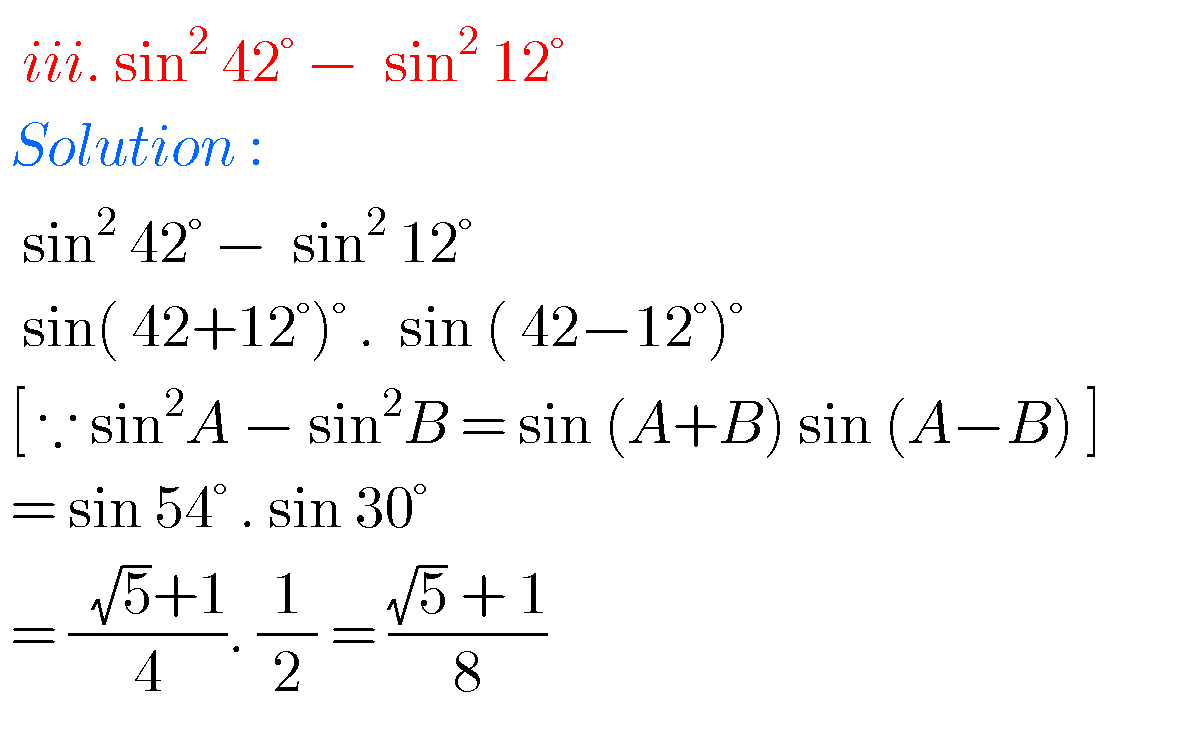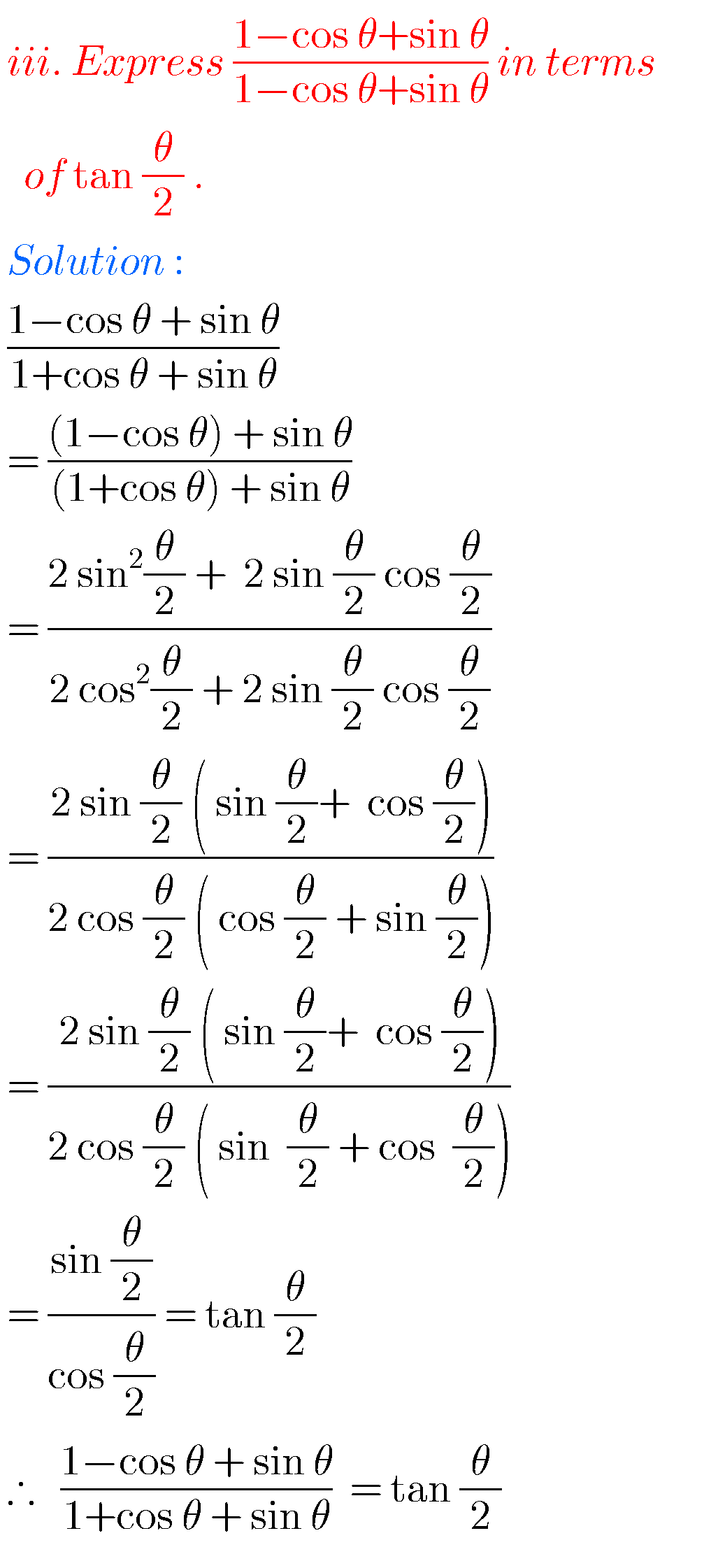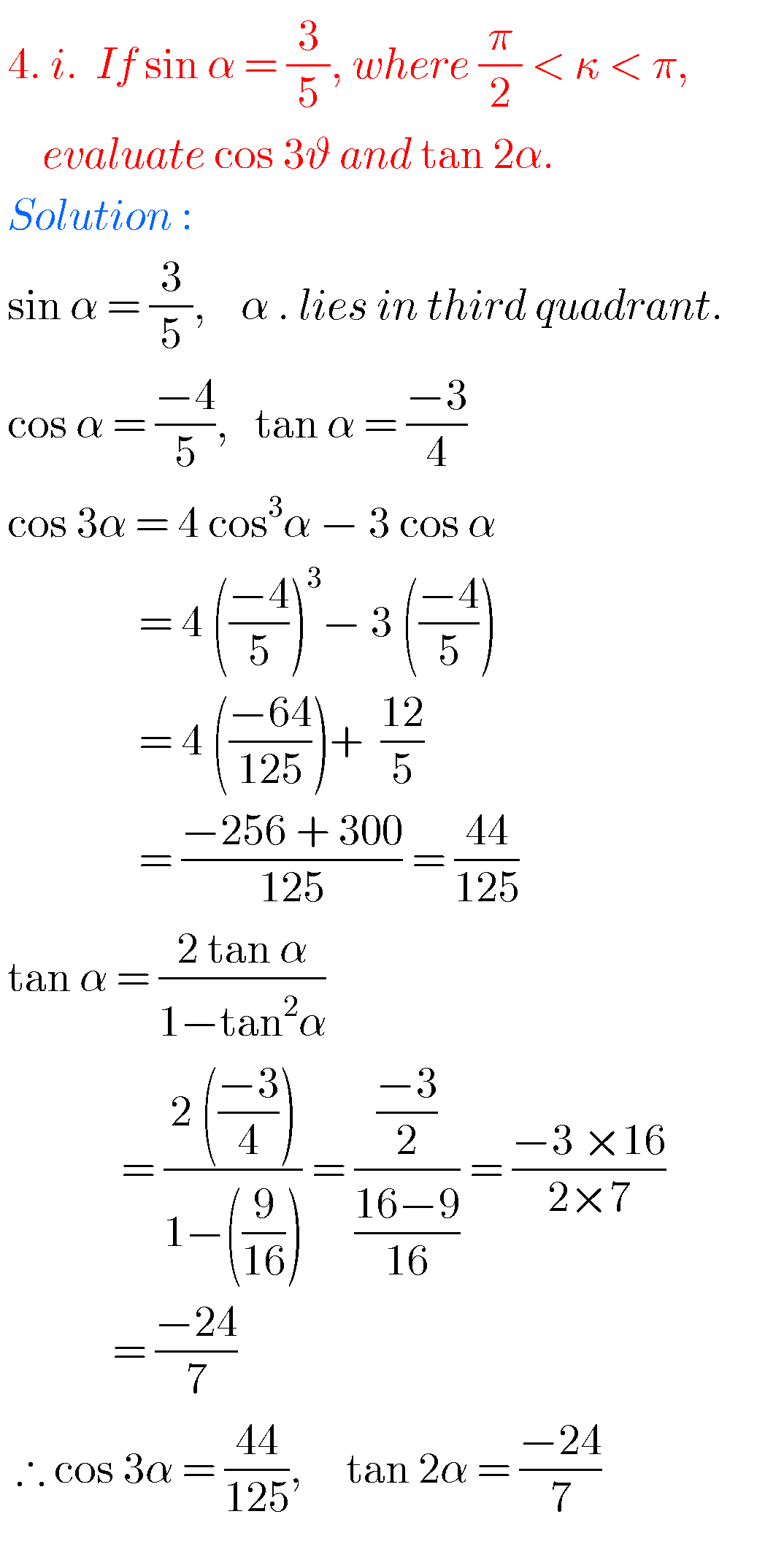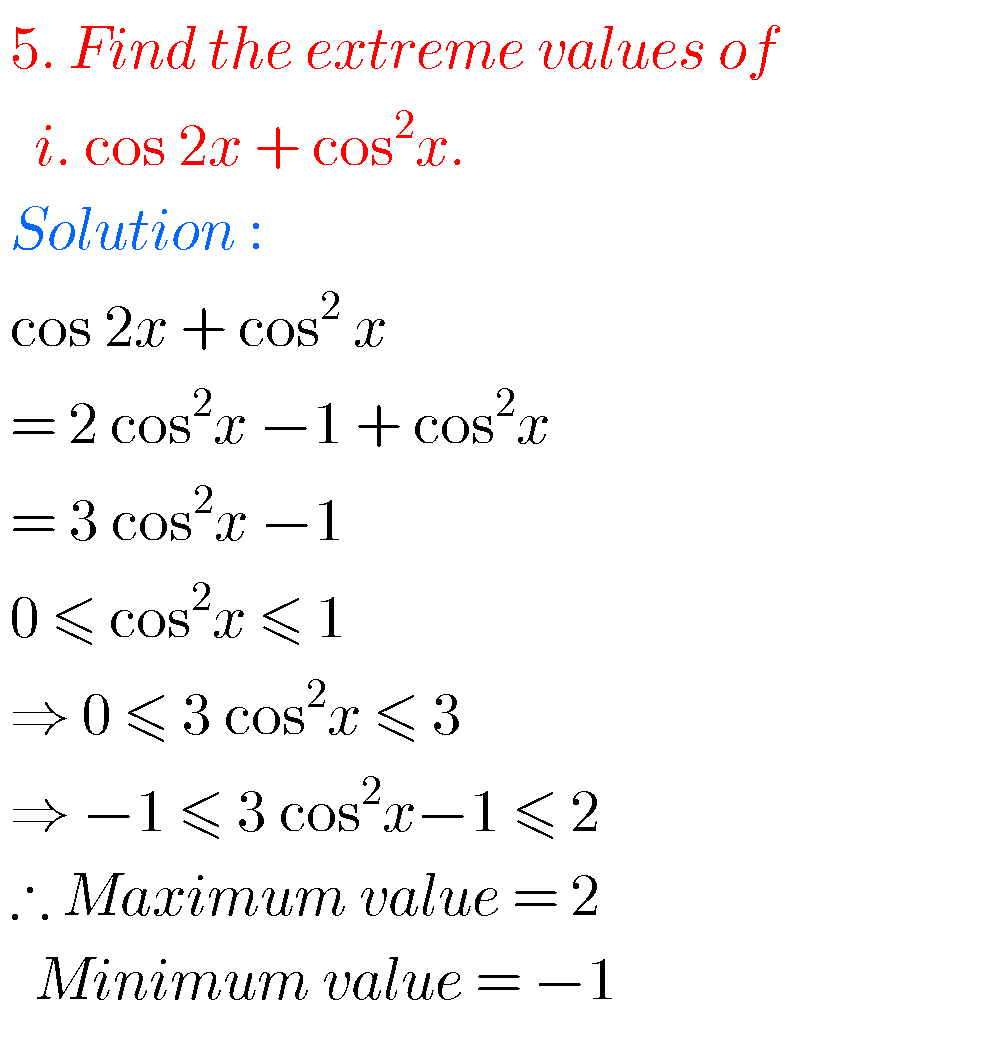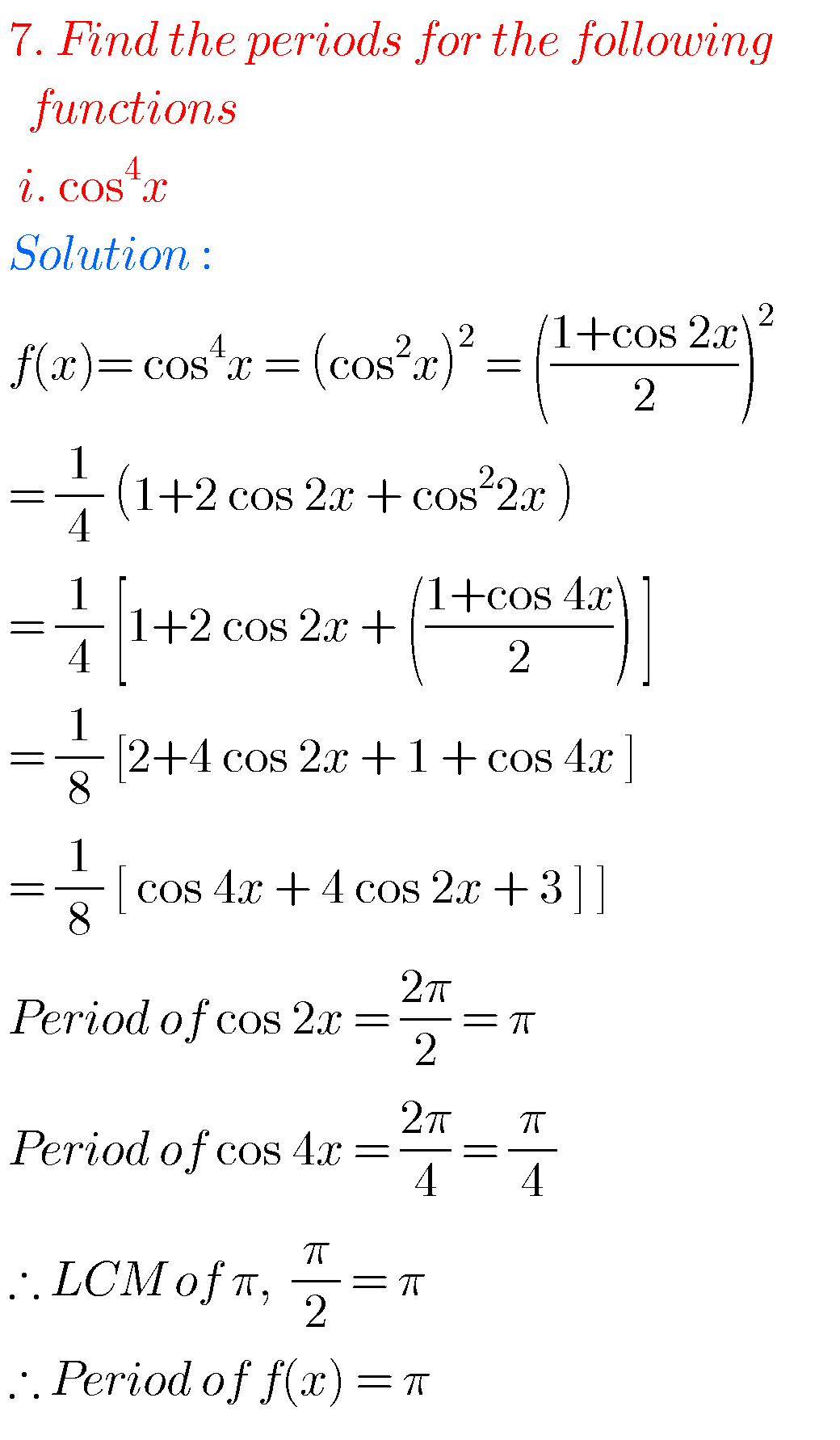## Trigonometric Ratios upto Transformations exercise 6(d) Solutions Inter Maths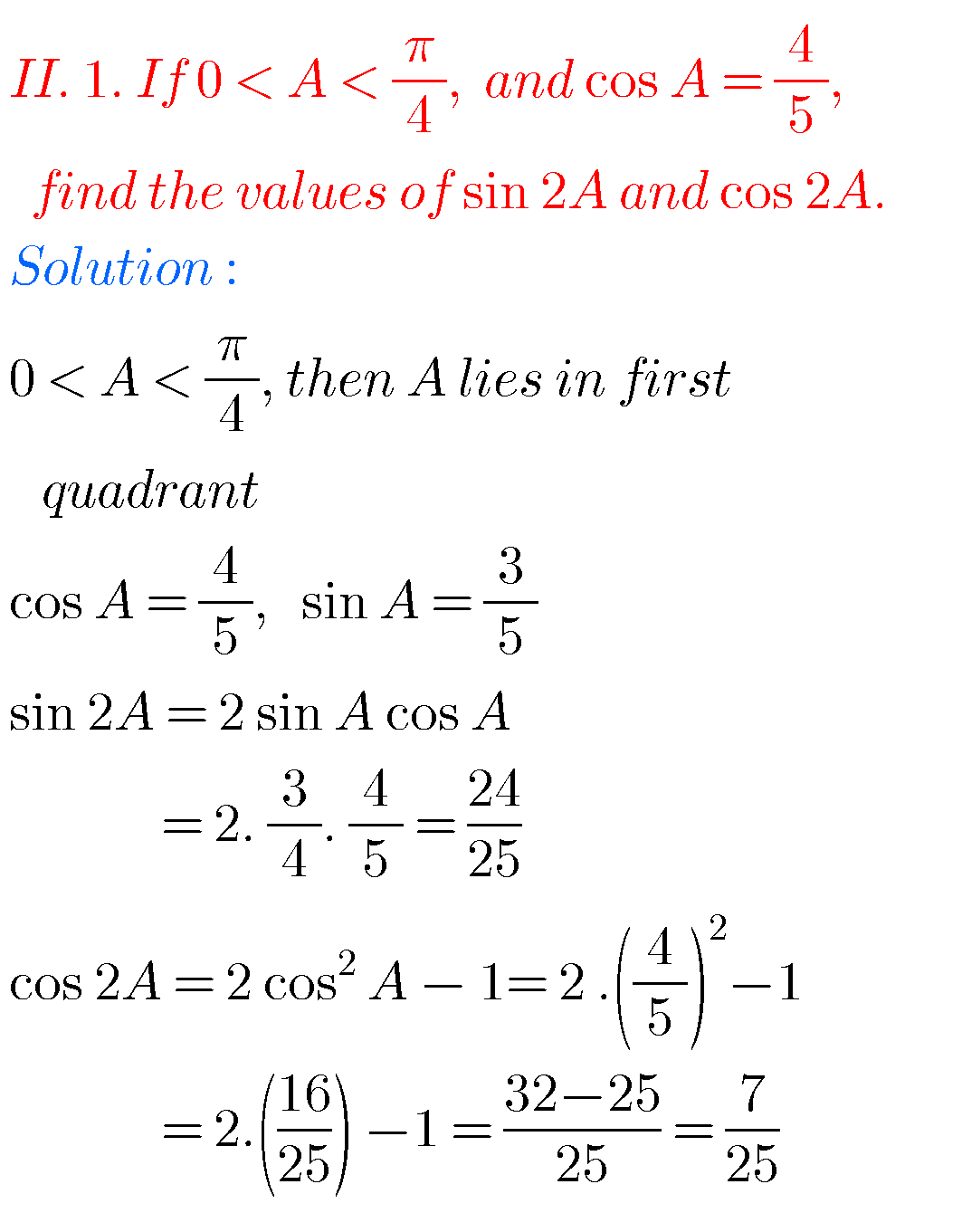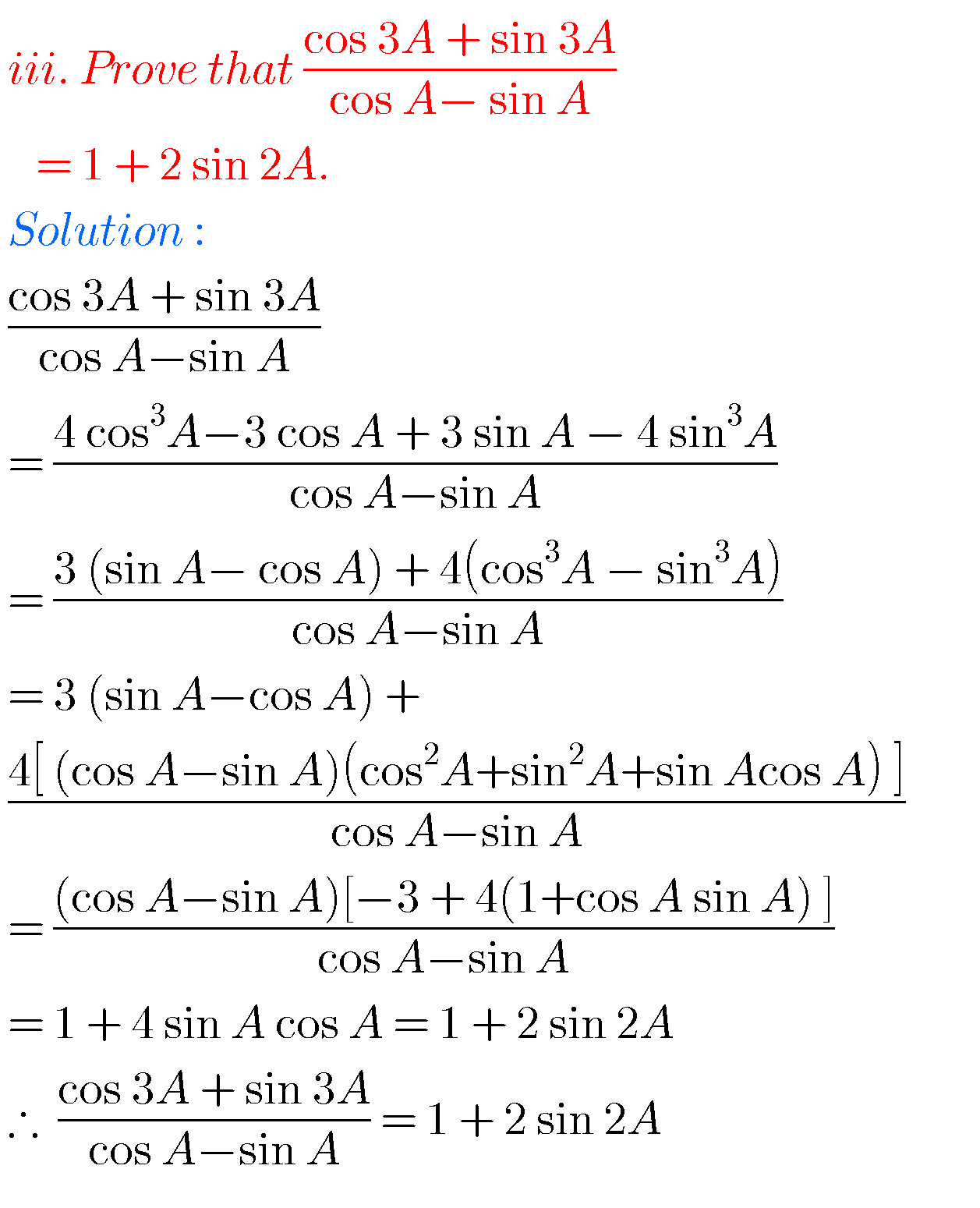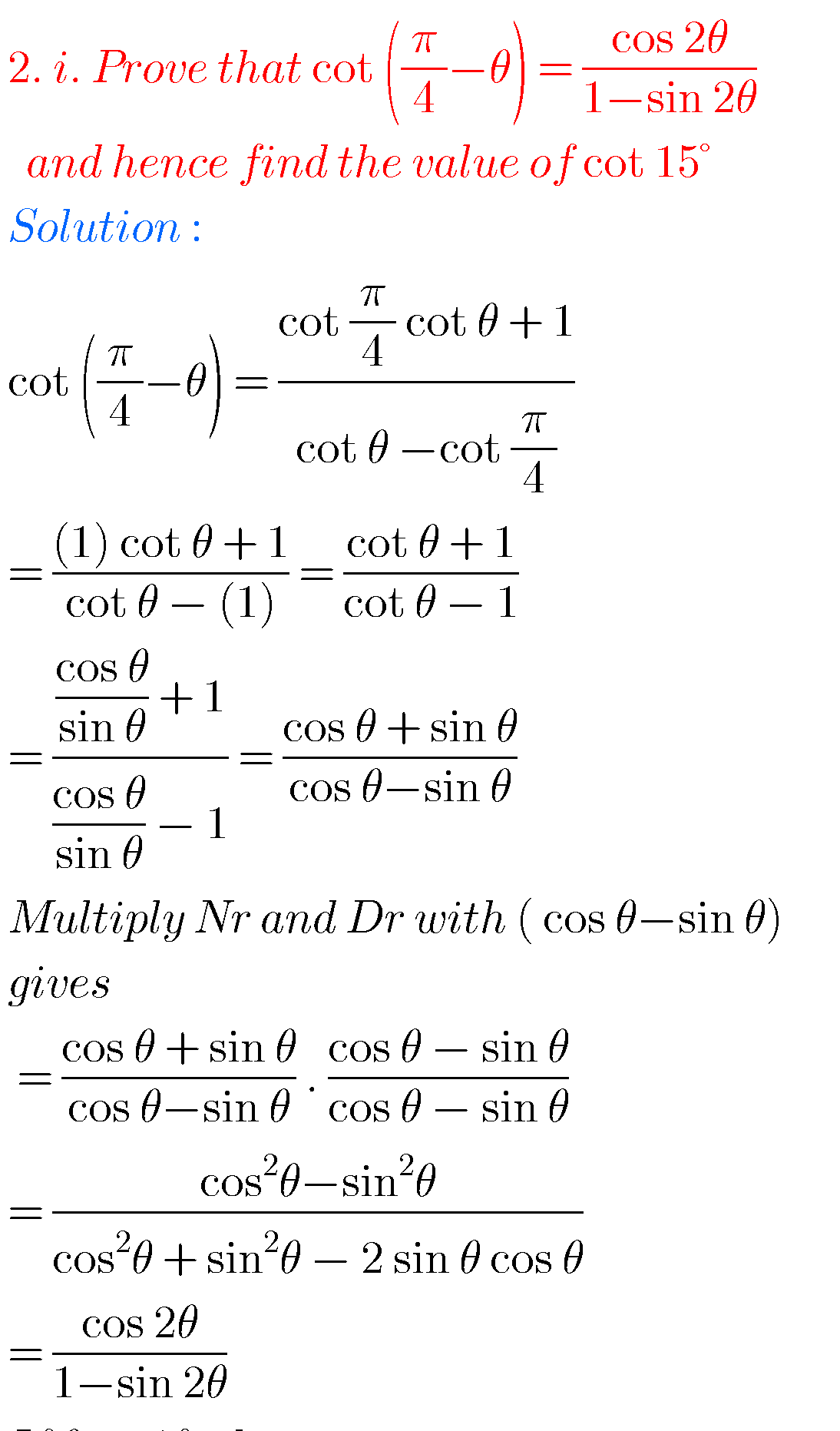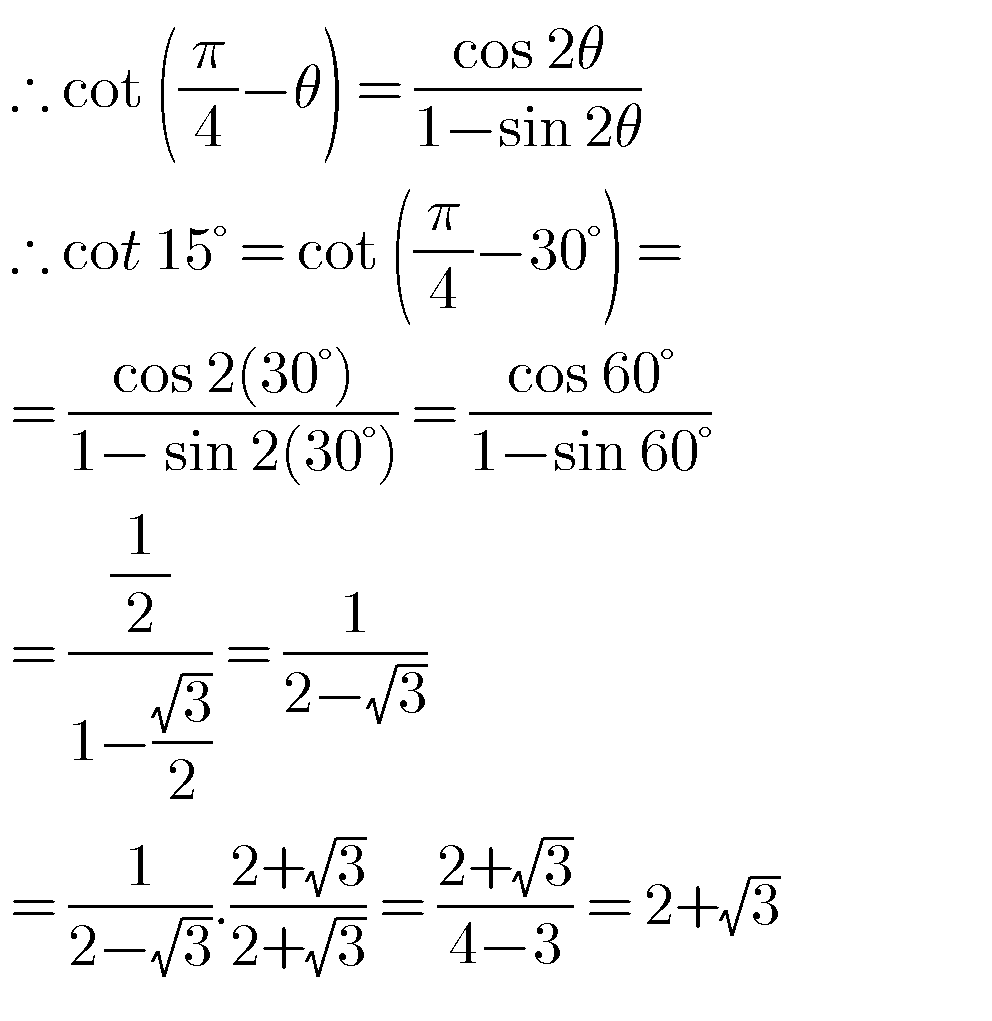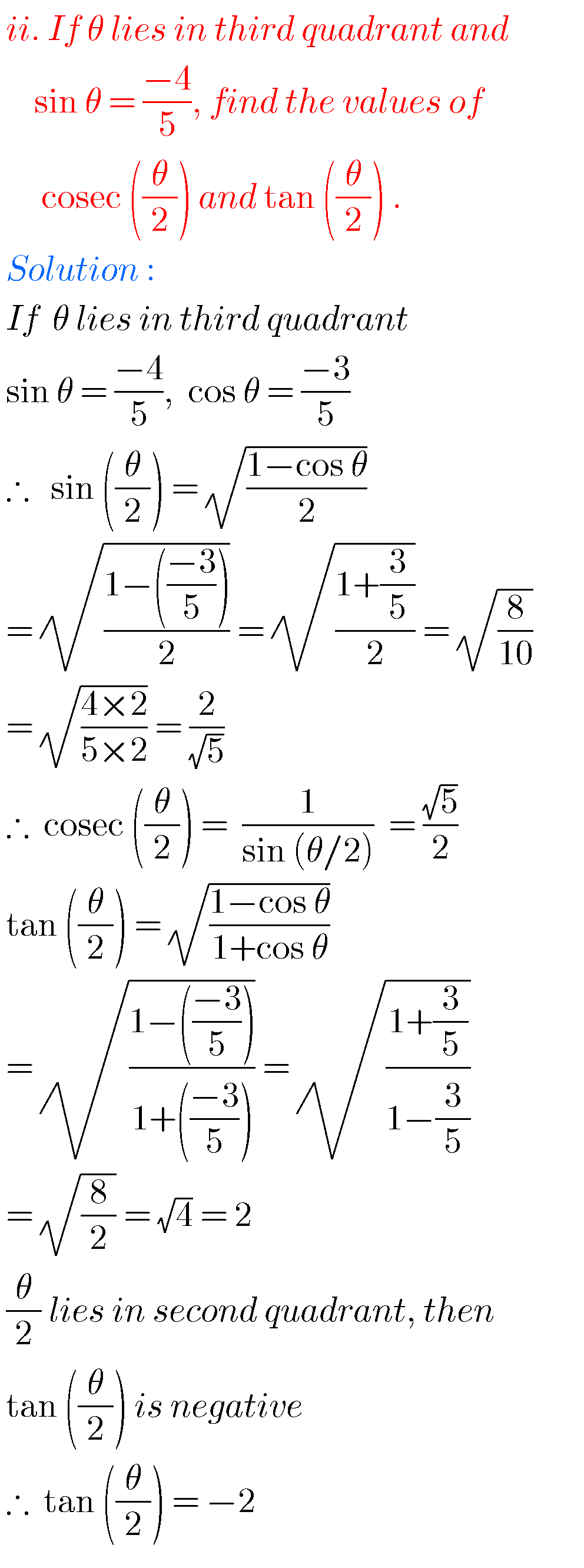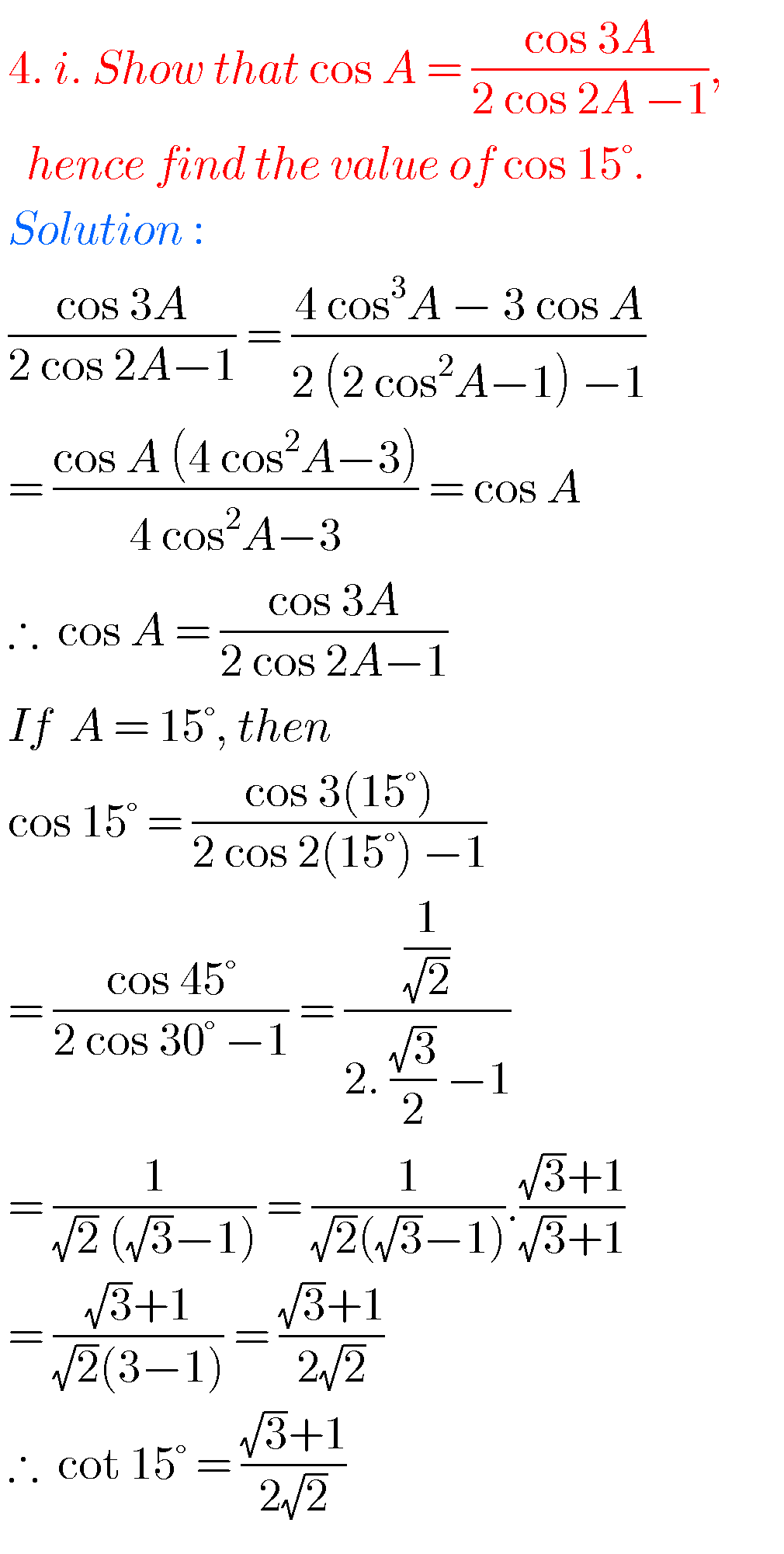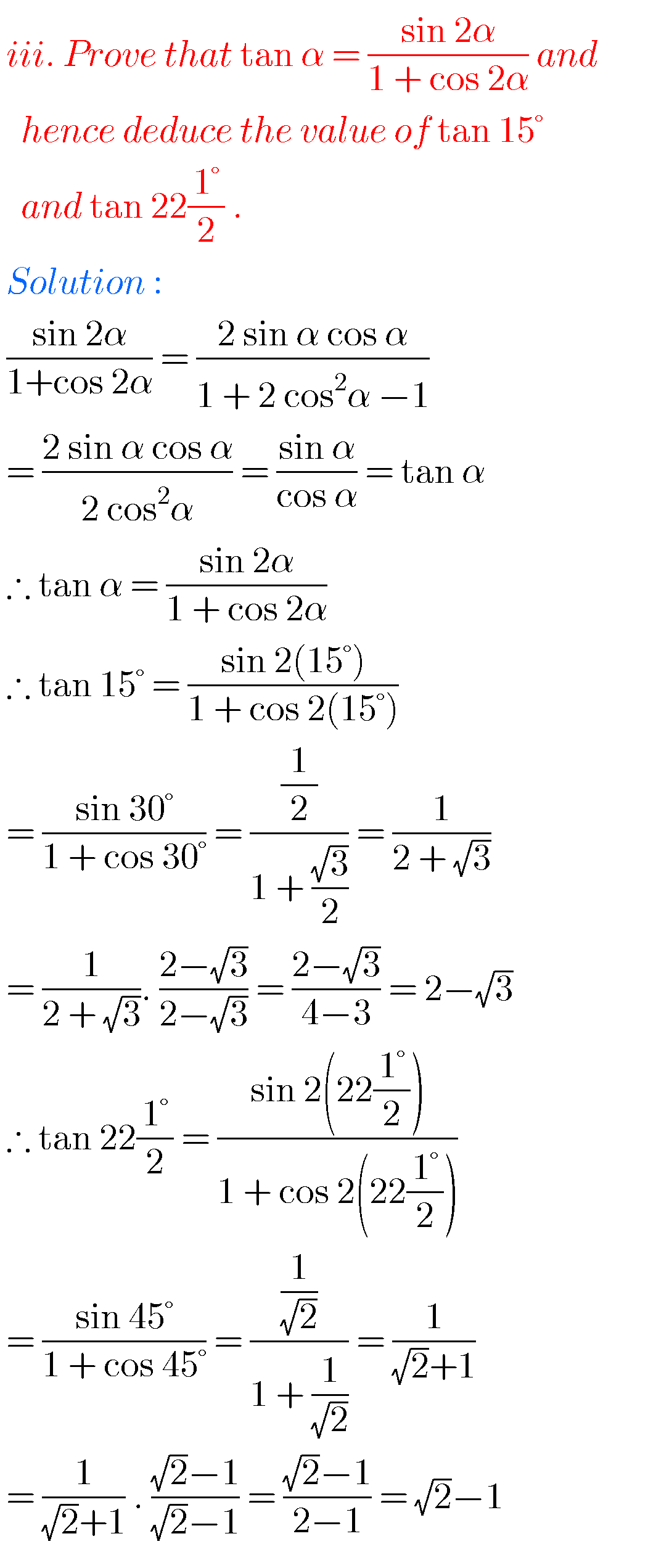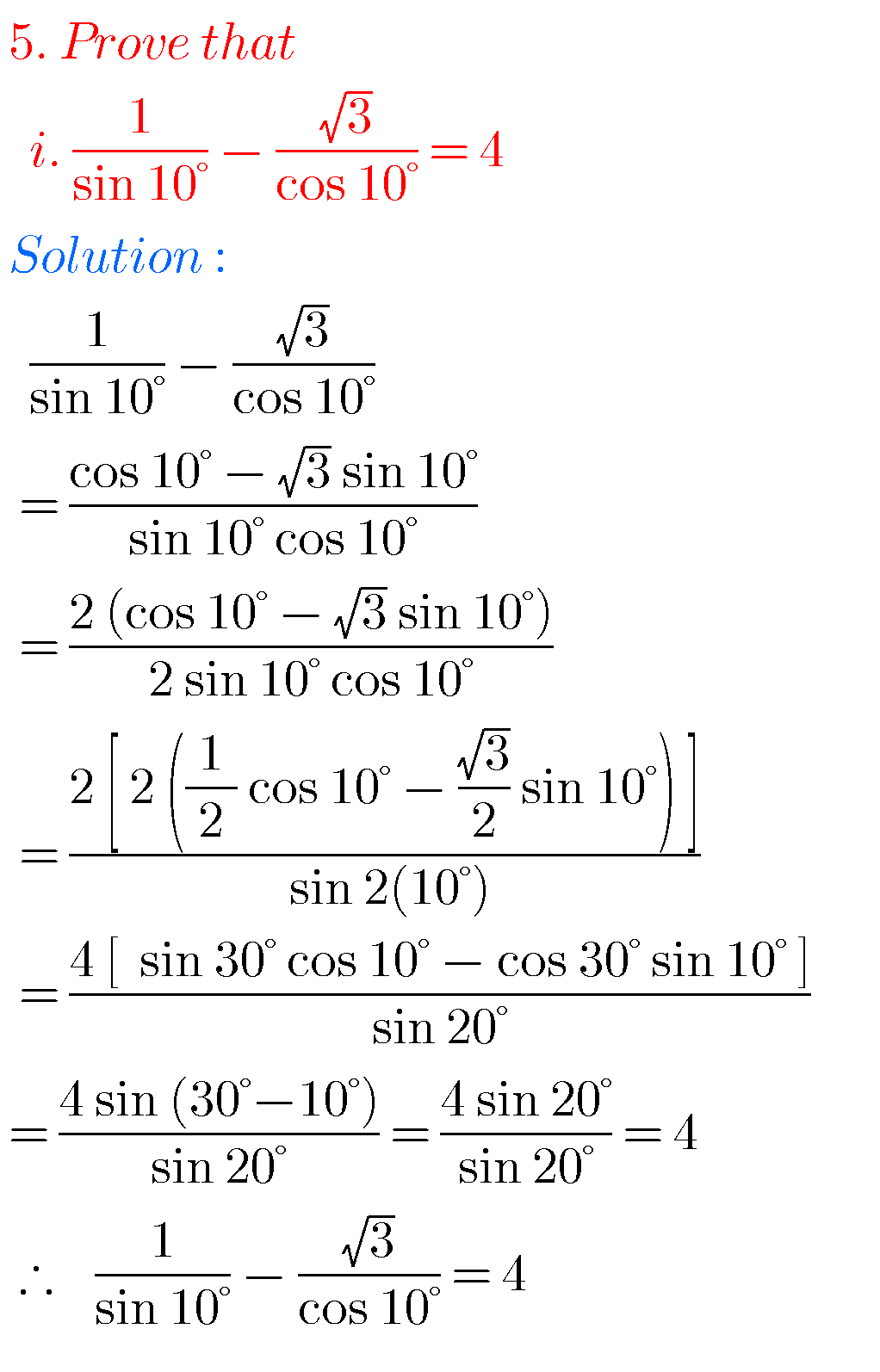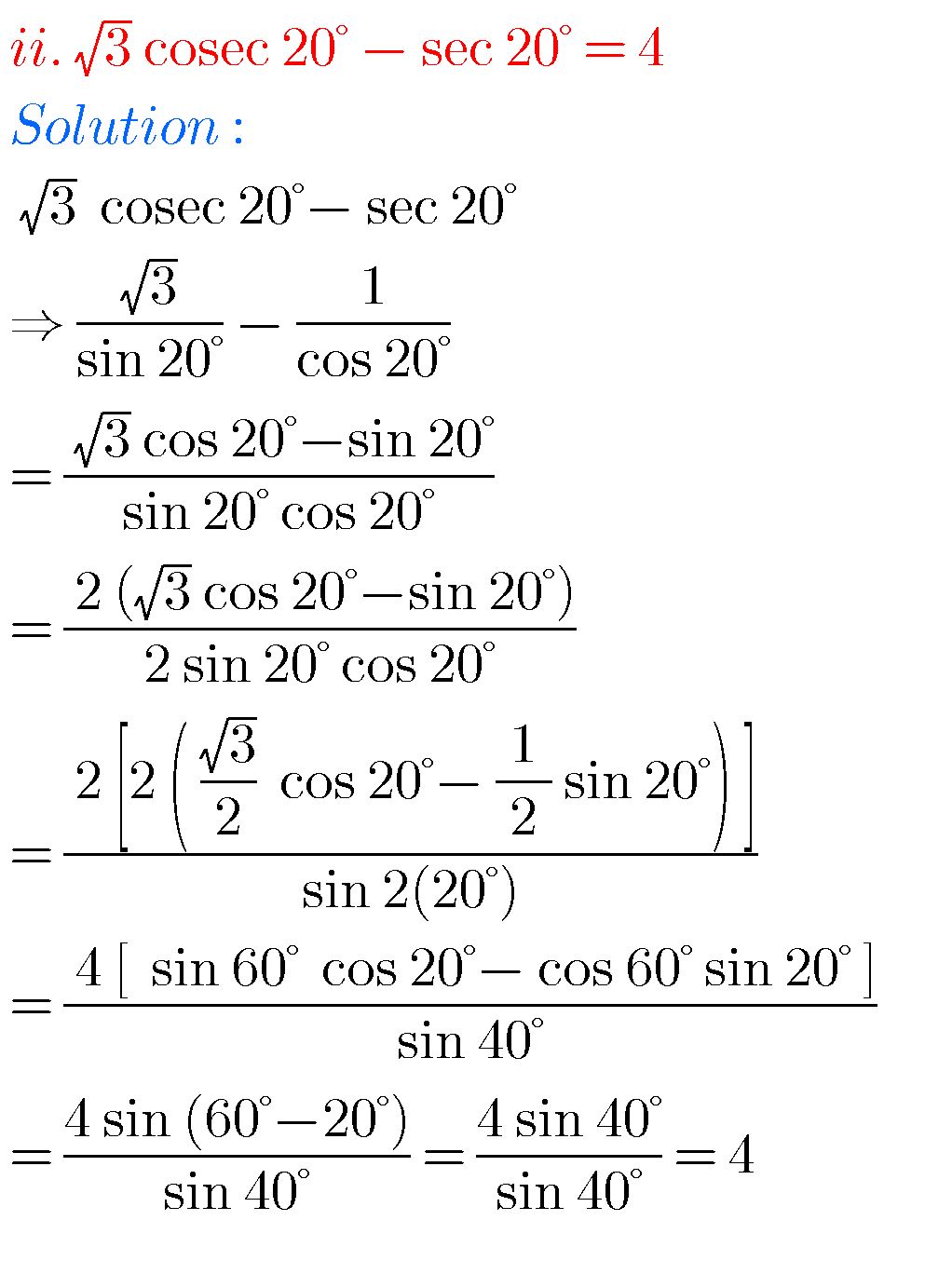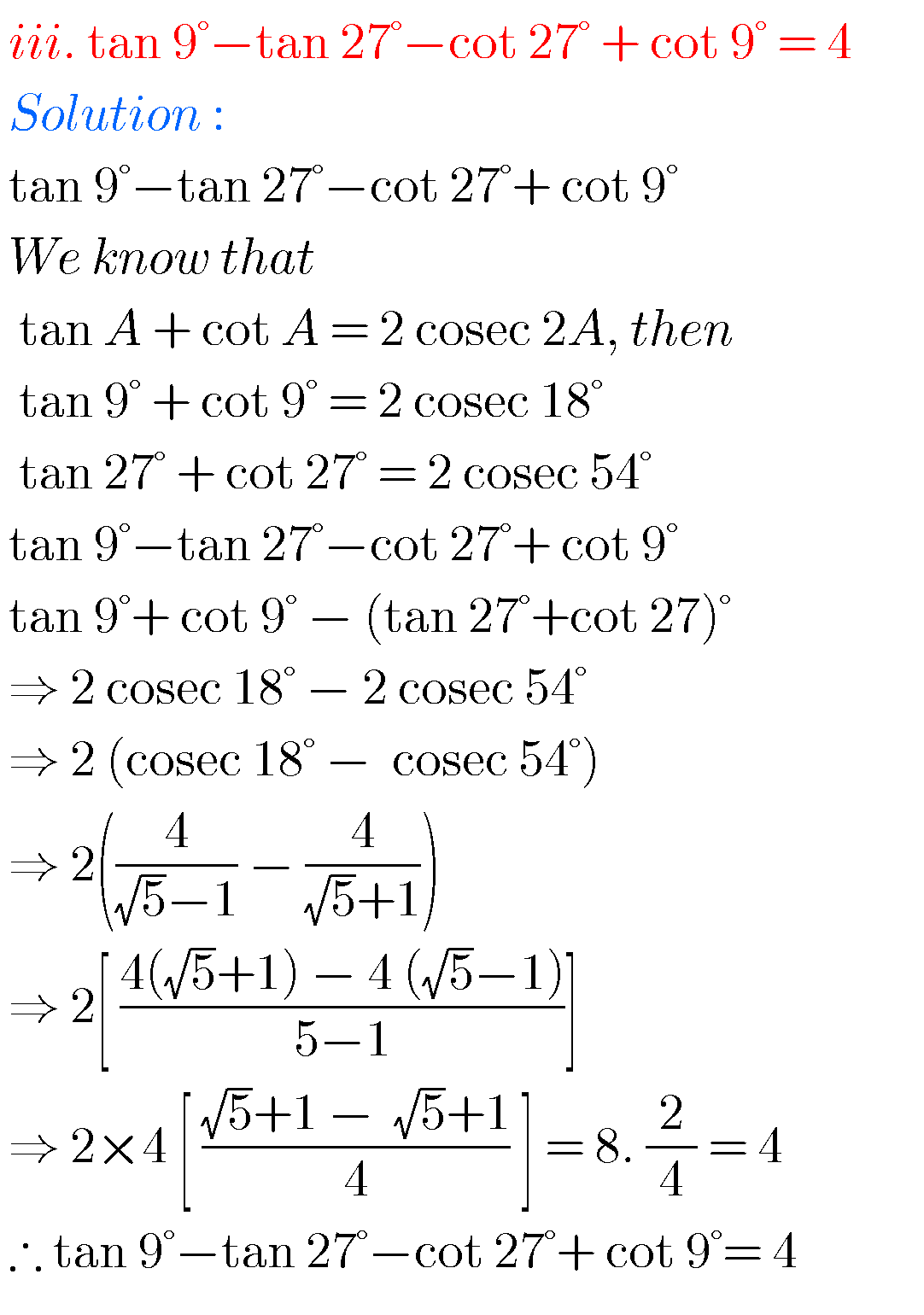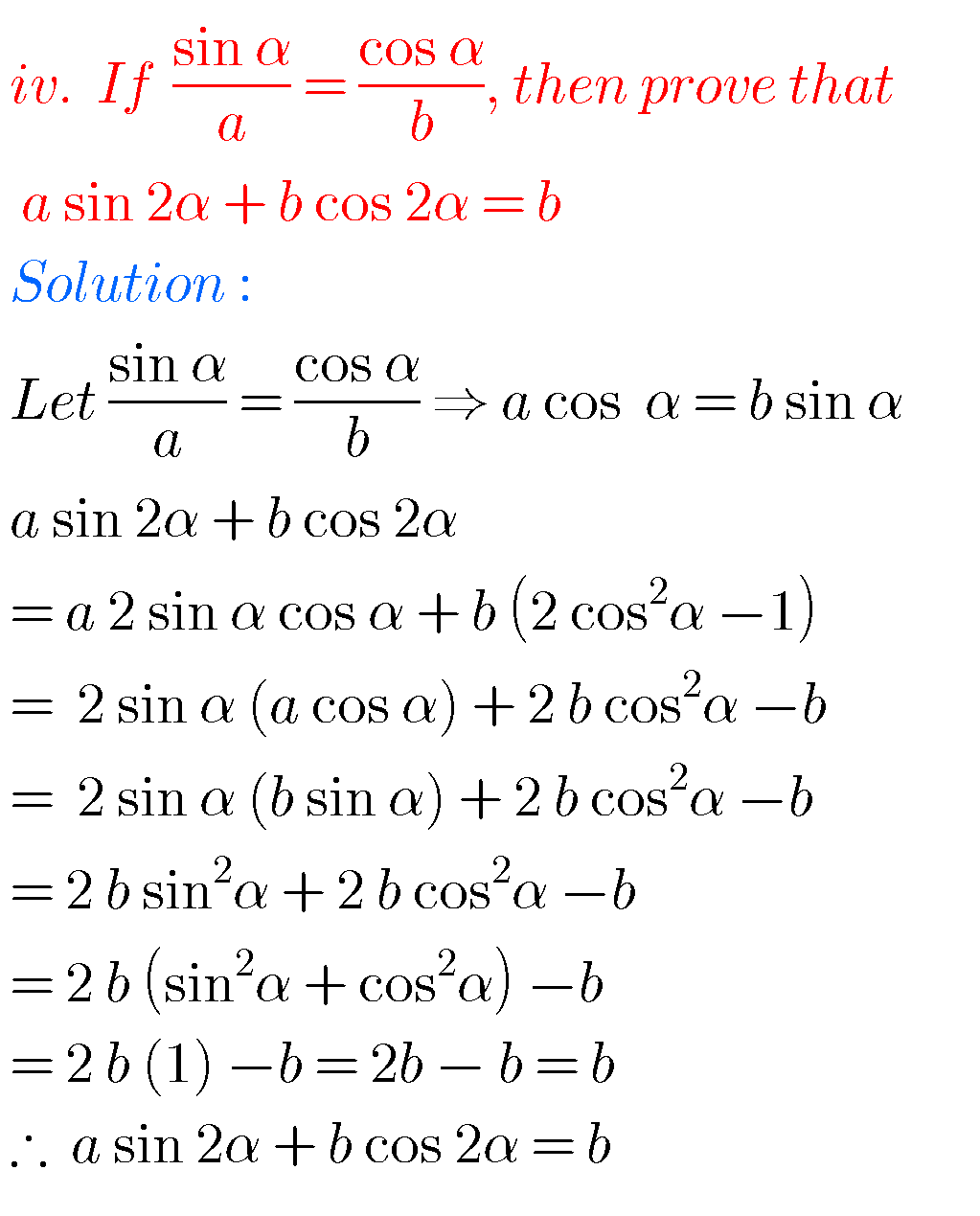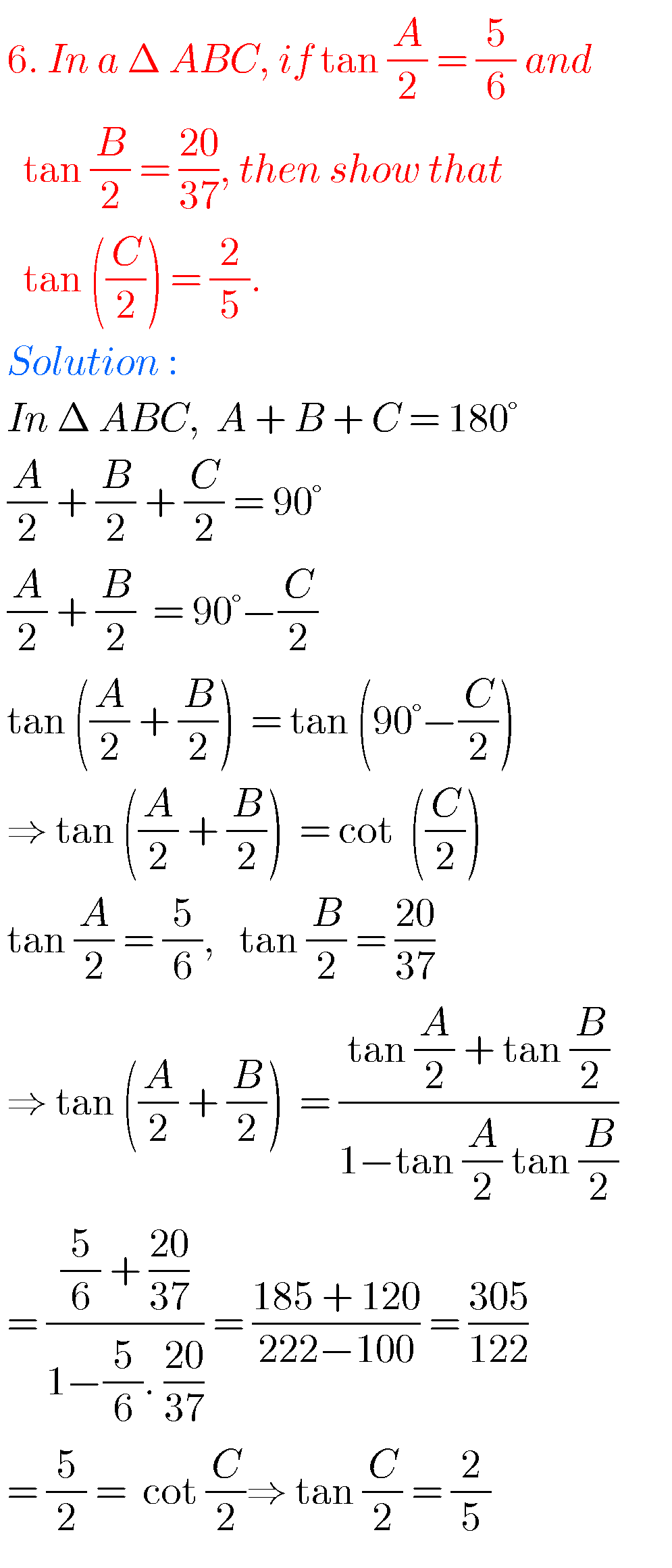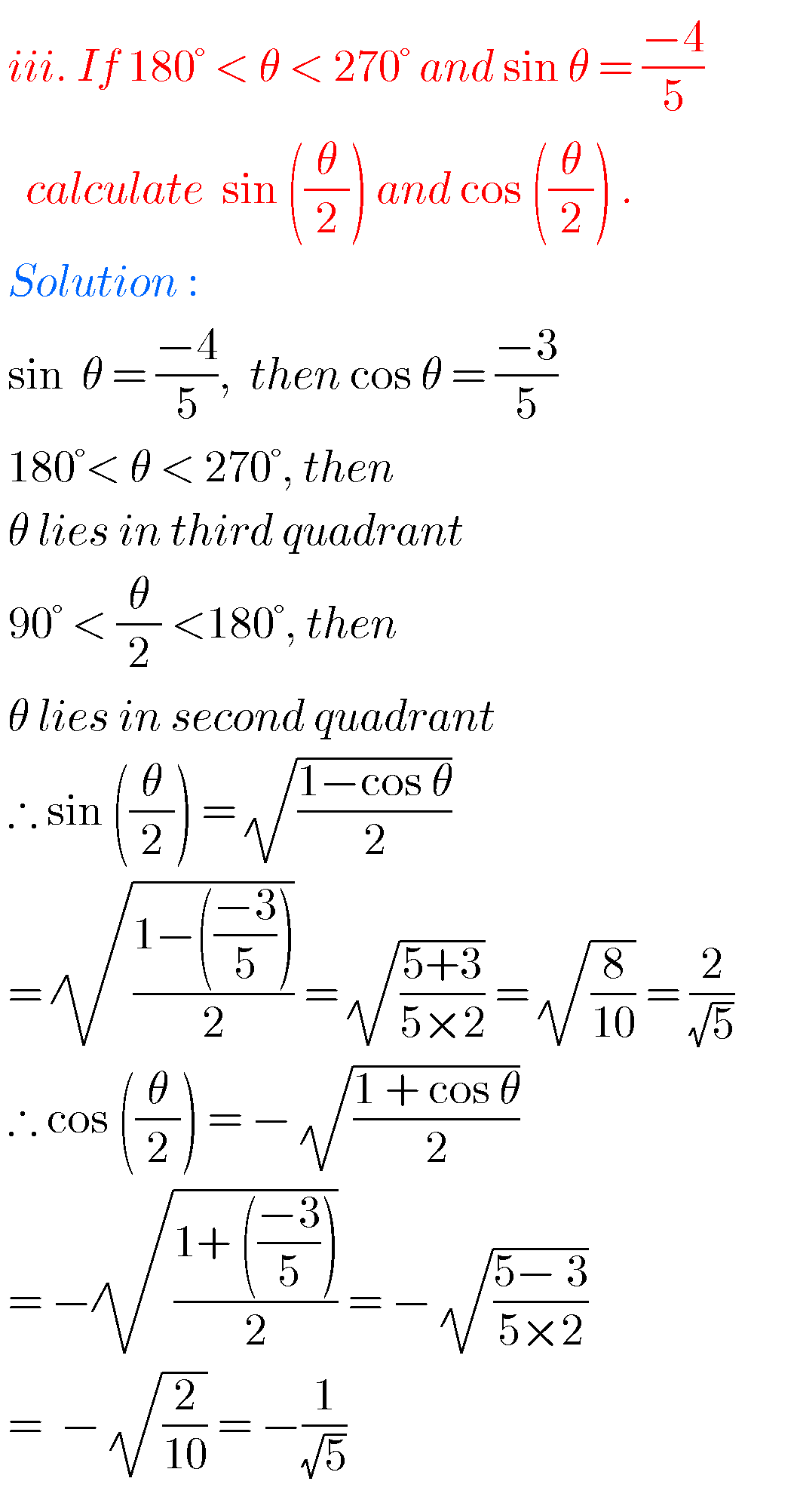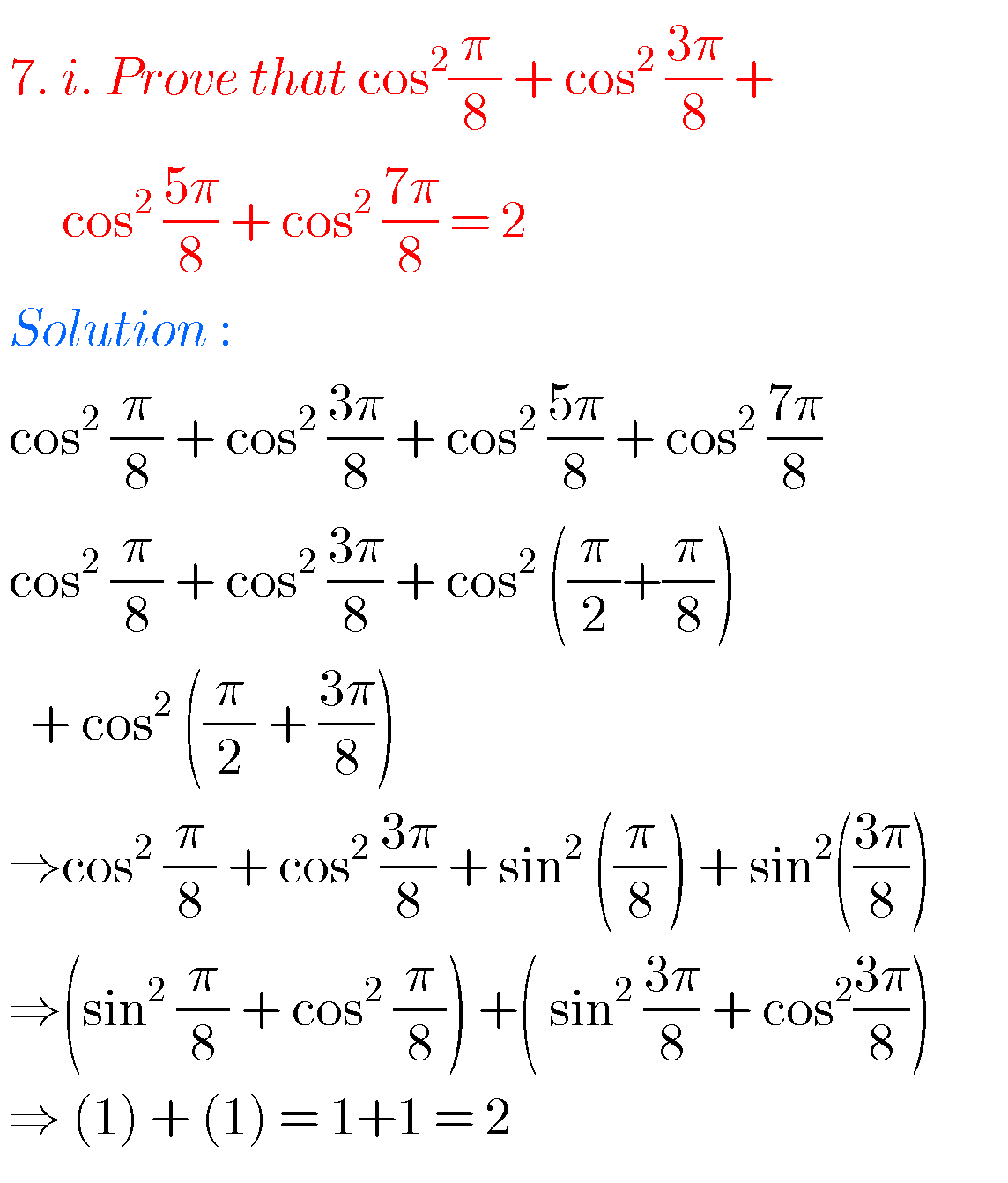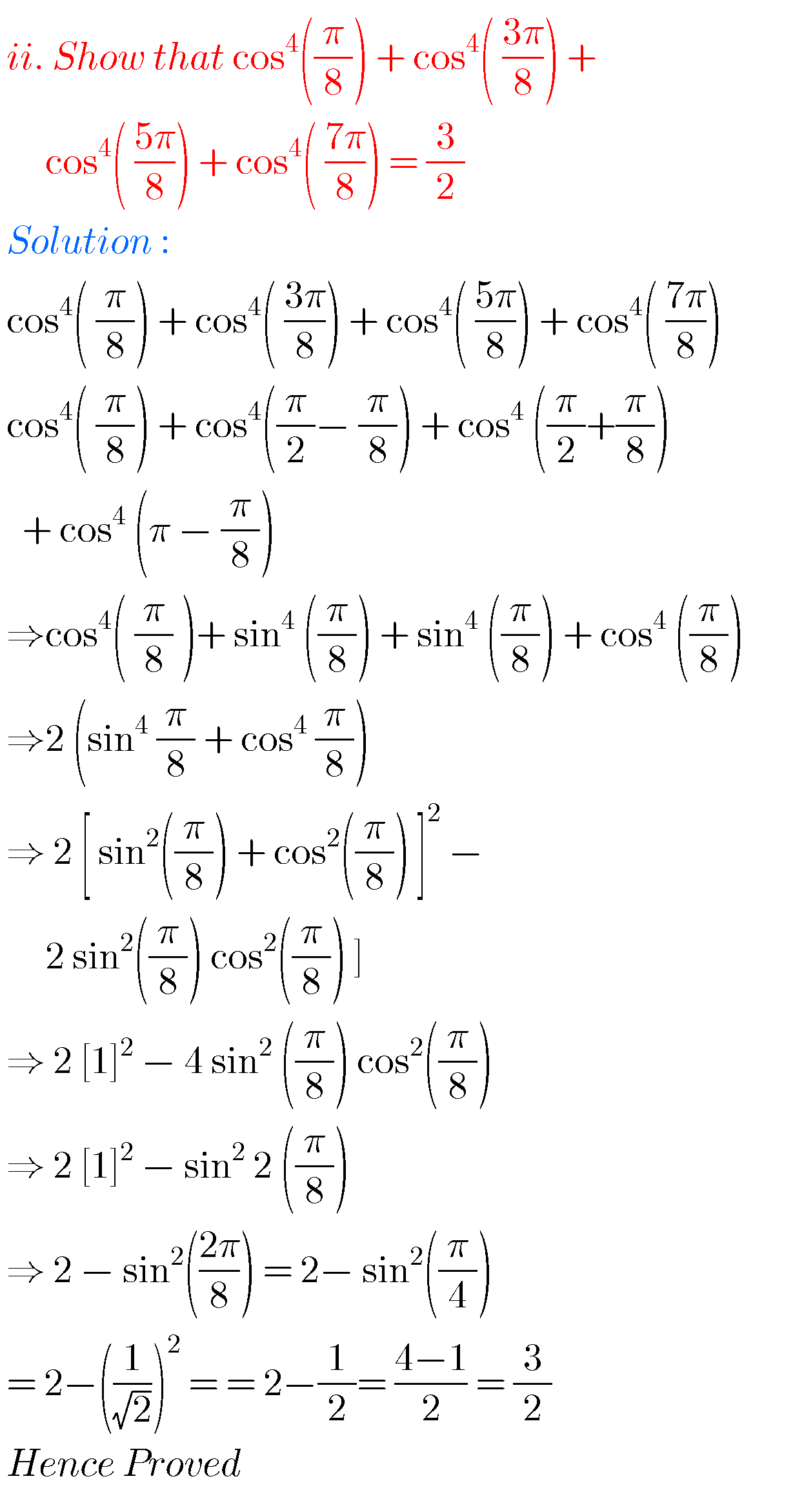### Maths 1A Solutions for Exercise 6(d) Inter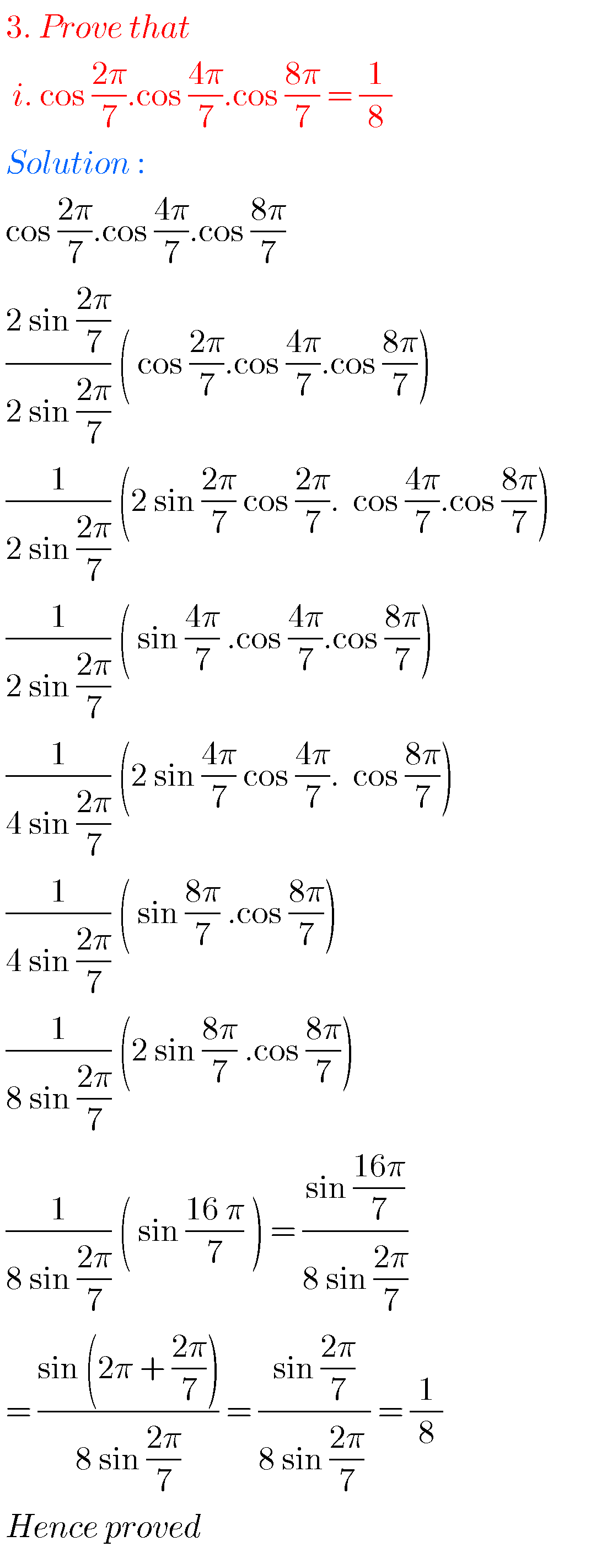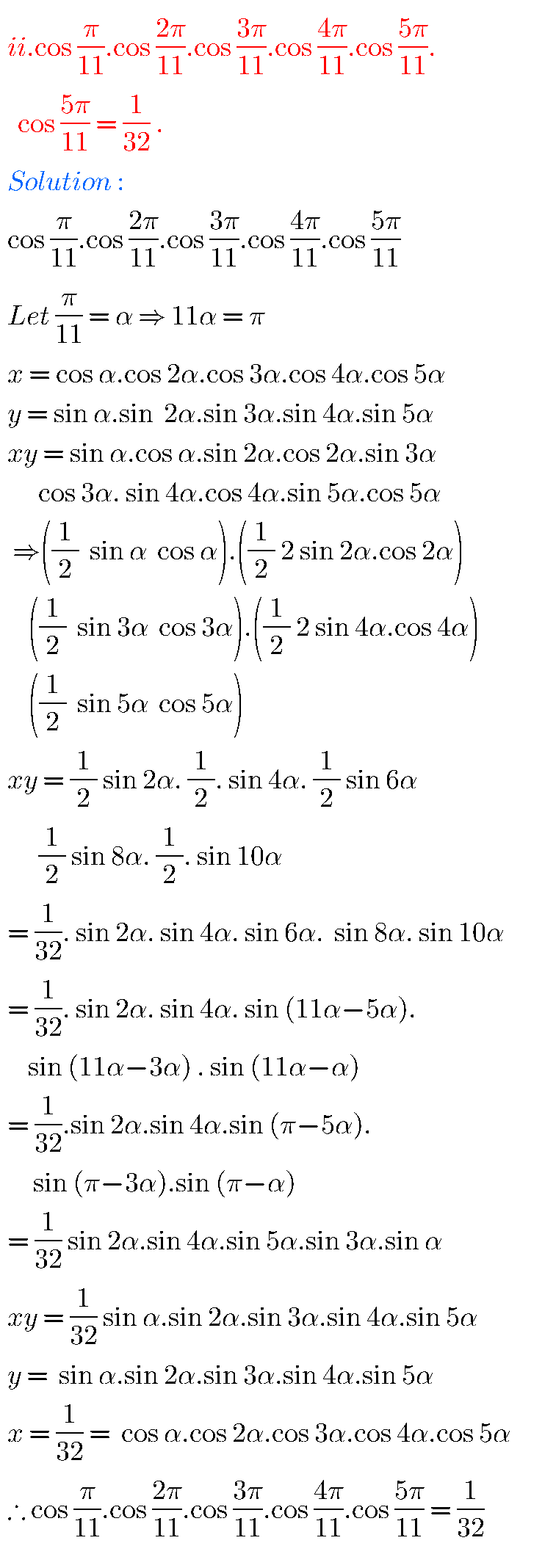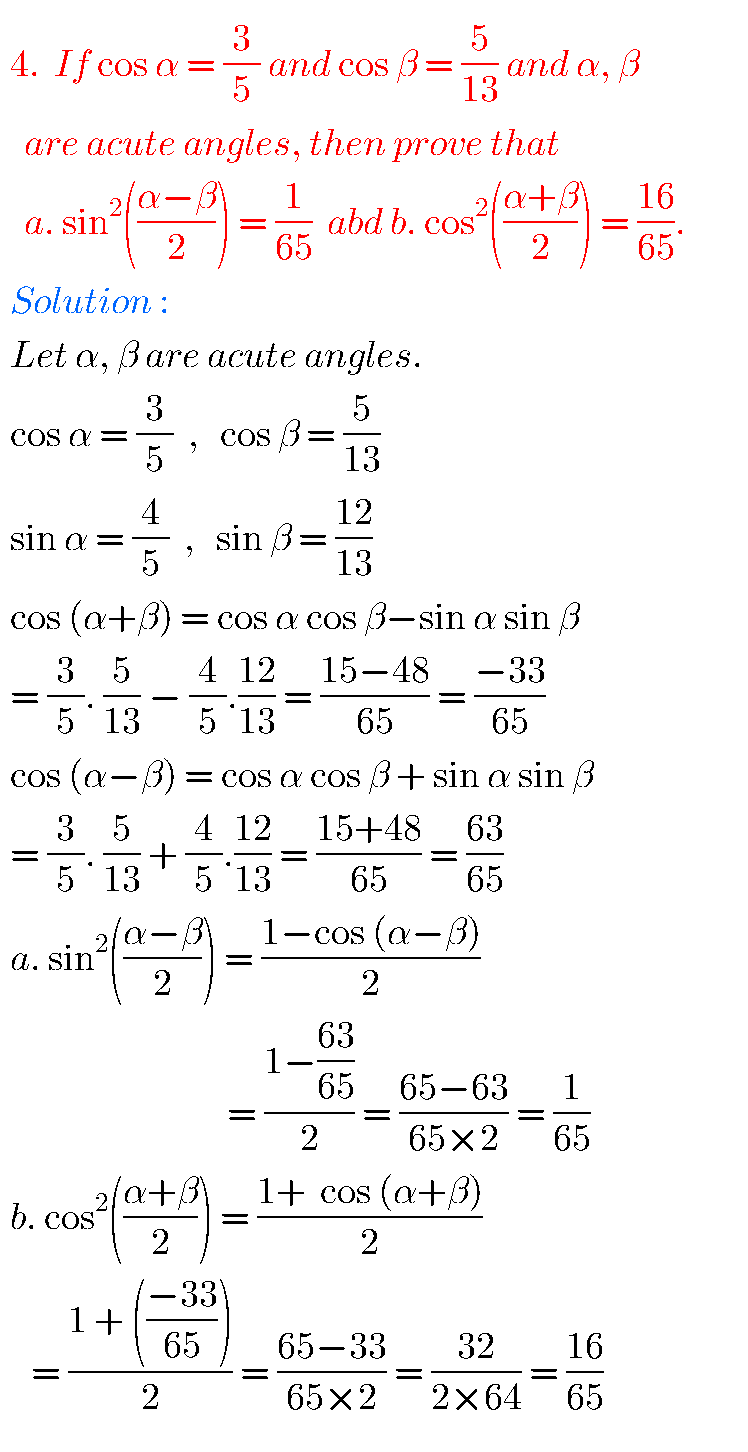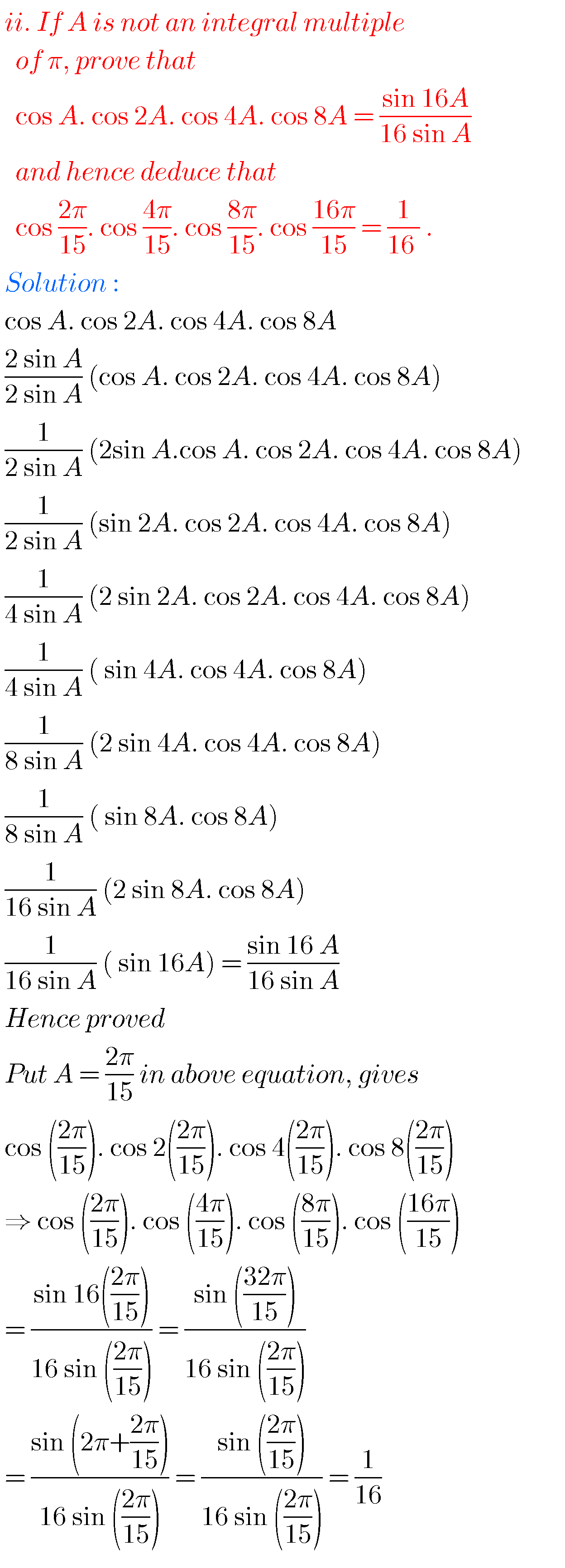Note : Observe the solutions and try them in your method.

SSC Maths Solutions

Ncert solutions for Principle of Mathematical Induction

Ncert solutions for Linear Equations in two variables class 8

Ncert solutions for Simple Equations class 7